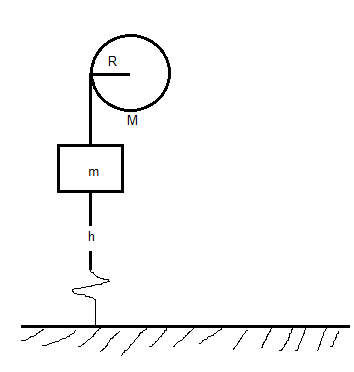In the system shown in the figure below, a m = 13.5 kg mass is released from rest and falls,...

Question:

In the system shown in the figure below, a {eq}m = 13.5\ kg {/eq} mass is released from rest and falls, causing the uniform {eq}M = 11.5\ kg {/eq} cylinder of diameter {eq}27.5\ cm {/eq} to turn about a frictionless axle through its center. How far will the mass have to descend to give the cylinder {eq}225\ J {/eq} of kinetic energy?Conservation Of Energy:

Energy is something that cannot be created by its own or can be destroyed by our own will but it can change itis from one form into another, for example, there are many forms of energy such as kinetic energy, potential energy, spring potential energy, etc.

Let for instance an apple is dropped from the table then the Potential energy stored in the apple before falling is converted into kinetic energy and will be maximum at the lowest point where potential energy is zero hence potential is not destroyed it is just converted into the kinetic energy.

Given:

• Mass of the object released is {eq}m=13.5\ kg {/eq}
• Mass of the cylinder is {eq}M=11.5\ kg {/eq}
• The diameter of the cylinder is {eq}D=27.5\ cm {/eq}
• Kinetic energy to be gain by cylinder is {eq}K.E=225\ J {/eq}

When the mass is released then the potential energy stored in the mass is converted into the kinetic energy of the mass Rotation kinetic energy of the cylinder.

Now from the conservation of energy:

{eq}P.E_i=K,E_f+R.E\\ mgh=\frac{1}{2}mv^2+\frac{1}{2}I\omega ^2 {/eq}

Here the height that is descended by the mass is {eq}h {/eq}

Moment of inertia for the cylinder is {eq}I=\frac{1}{2}MR^2 {/eq}

Angular velocity of the cylinder is {eq}\omega =\frac{v}{R} {/eq}

For the final velocity of the mass

{eq}R.E=\frac{1}{2}I\omega^2\\ R.E=\frac{1}{2}\times \frac{1}{2}MR^2\times \frac{v^2}{R^2}\\ R.E=\frac{1}{2}Mv^2 {/eq}

Since the rotational kinetic energy of the cylinder has to be equal to 225 J:

{eq}225=\frac{1}{2}Mv^2\\ 225=\frac{1}{2}\times (11.5)v^2\\ 225=5.75\ v^2\\ v^2=39.13\\ v=6.25\ m/s {/eq}

Thus, the velocity by which the mass is descending downward is 6.25 m/s

Now for the height descended by the mass:

{eq}mgh=\frac{1}{2}mv^2+\frac{1}{2}I\omega ^2\\ (13.5)\times 9.81\times h=\frac{1}{2}(13.5)\times (6.25)^2+225\\ 132.43\ h=263.67+225\\ 132.43\ h=488.67\\ h=3.69\ m {/eq}

Thus, the height by which the mass is descended is 3.69 m